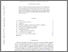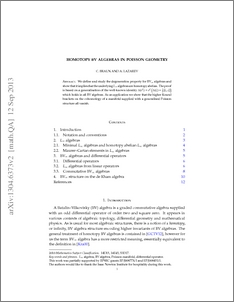# Homotopy BV algebras in Poisson geometry

Braun, Christopher and Lazarev, Andrey (2013) Homotopy BV algebras in Poisson geometry. Transactions of Moscow Mathematical Society, 74 (2). pp. 217-227. ISSN 0077-1554Preview
PDF
1304.6373v2.pdf - Accepted Version

We define and study the degeneration property for $\mathrm {BV}_\infty$ algebras and show that it implies that the underlying $L_{\infty }$ algebras are homotopy abelian. The proof is based on a generalisation of the well-known identity $\Delta (e^{\xi })=e^{\xi }\Big (\Delta (\xi )+\frac {1}{2}[\xi ,\xi ]\Big )$ which holds in all BV algebras. As an application we show that the higher Koszul brackets on the cohomology of a manifold supplied with a generalised Poisson structure all vanish. - See more at: http://www.ams.org/journals/mosc/2013-74-00/S0077-1554-2014-00216-8/#sthash.pBIIcZKa.dpufView Item